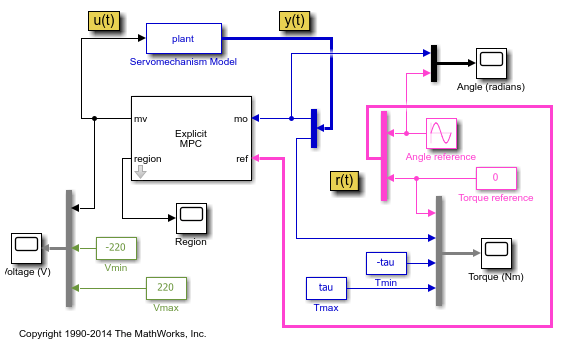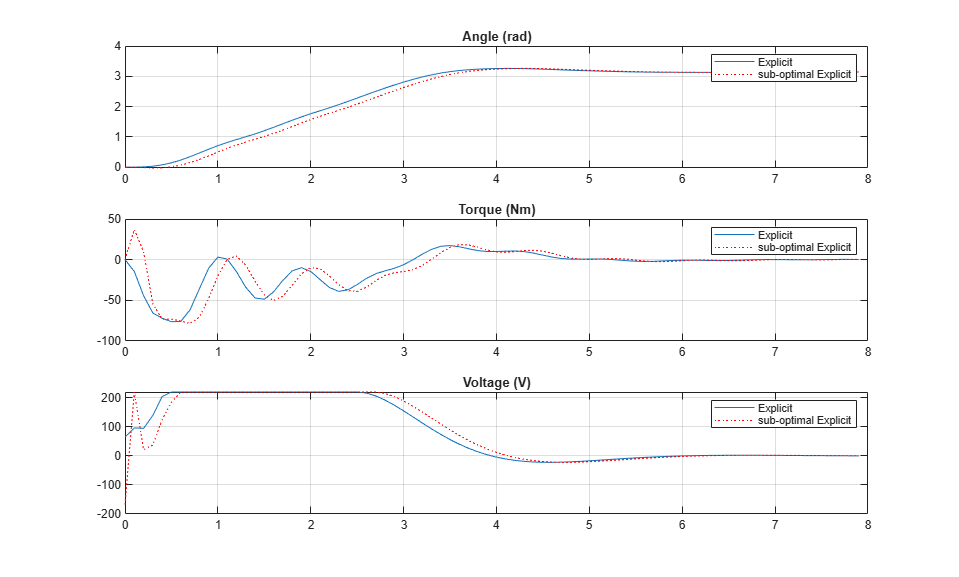Explicit MPC Control of DC Servomotor with Constraint on Unmeasured Output

This example shows how to use explicit MPC to control a DC servomechanism under voltage and shaft torque constraints.

For a similar example that uses traditional implicit MPC, see DC Servomotor with Constraint on Unmeasured Output.

Define DC-Servo Motor Model

The linear open-loop dynamic model is defined in plant. Variable tau is the maximum admissible torque to be used as an output constraint.

[plant,tau] = mpcmotormodel;

Specify input and output signal types for the MPC controller. The second output, torque, is unmeasurable.

plant = setmpcsignals(plant,'MV',1,'MO',1,'UO',2);

Specify Constraints

The manipulated variable is constrained between +/- 220 volts. Since the plant inputs and outputs are of different orders of magnitude, you also use scale factors to facilitate MPC tuning. Typical choices of scale factor are the upper/lower limit or the operating range.

MV = struct('Min',-220,'Max',220,'ScaleFactor',440);

Torque output constraints are only imposed during the first three prediction steps to limit the complexity of the explicit MPC design.

OV = struct('Min',{Inf, [-tau;-tau;-tau;-Inf]},...
'Max',{Inf, [tau;tau;tau;Inf]},...
'ScaleFactor',{2*pi, 2*tau});

Specify Tuning Weights

The control task is to get zero tracking offset for the angular position. Since you only have one manipulated variable, the shaft torque is allowed to float within its constraint by setting its weight to zero.

Weights = struct('MV',0,'MVRate',0.1,'OV',[0.1 0]);

Create MPC controller

Create an MPC controller with sample time Ts, prediction horizon p, and control horizon m.

Ts = 0.1;
p = 10;
m = 2;
mpcobj = mpc(plant,Ts,p,m,Weights,MV,OV);

Generate Explicit MPC Controller

Explicit MPC executes the equivalent explicit piecewise affine version of the MPC control law defined by the traditional implicit MPC controller. To generate an explicit MPC controller from an implicit MPC controller, you must specify the range for each controller state, reference signal, manipulated variable, and measured disturbance so that the multi-parametric quadratic programming problem is solved in the parameter sets defined by these ranges.

Create a range structure where you can specify the range for each parameter afterwards.

range = generateExplicitRange(mpcobj);
-->Converting model to discrete time.
Assuming no disturbance added to measured output channel #1.
-->The "Model.Noise" property of the "mpc" object is empty. Assuming white noise on each measured output channel.

Specify ranges for controller states

MPC controller states include states from the plant model, disturbance model, and noise model in that order. Setting the range of a state variable is sometimes difficult when the state does not correspond to a physical parameter. In that case, to collect state range data, running multiple open-loop plant simulations with typical reference and disturbance signals is recommended.

range.State.Min(:) = -1000;
range.State.Max(:) = 1000;

Usually you know the practical range of the reference signals being used at the nominal operating point in the plant. The ranges used to generate the explicit MPC controller must be at least as large as the practical range. Note that the range for torque reference is fixed at 0 because it has zero weight.

range.Reference.Min = [-5;0];
range.Reference.Max = [5;0];

If manipulated variables are constrained, the ranges used to generate the explicit MPC controller must be at least as large as these limits.

range.ManipulatedVariable.Min = MV.Min - 1;
range.ManipulatedVariable.Max = MV.Max + 1;

Create an explicit MPC controller with the specified ranges.

mpcobjExplicit = generateExplicitMPC(mpcobj,range)

Regions found / unexplored:       75/       0

Explicit MPC Controller
---------------------------------------------
Controller sample time:    0.1 (seconds)
Polyhedral regions:        75
Number of parameters:      6
Is solution simplified:    No
State Estimation:          Default Kalman gain
---------------------------------------------
Type 'mpcobjExplicit.MPC' for the original implicit MPC design.
Type 'mpcobjExplicit.Range' for the valid range of parameters.
Type 'mpcobjExplicit.OptimizationOptions' for the options used in multi-parametric QP computation.
Type 'mpcobjExplicit.PiecewiseAffineSolution' for regions and gain in each solution.

Plot Piecewise Affine Partition

You can review any 2-D section of the piecewise affine partition defined by the explicit MPC control law. To do so, first create a parameter structure where you can specify which 2-D section to plot.

params = generatePlotParameters(mpcobjExplicit);

In this example, you plot the first state variable against the second state variable. All the other parameters must be fixed at values within their respective ranges.

Fix other state variables.

params.State.Index = [3 4];
params.State.Value = [0 0];

Fix reference signals.

params.Reference.Index = [1 2];
params.Reference.Value = [pi 0];

Fix manipulated variables.

params.ManipulatedVariable.Index = 1;
params.ManipulatedVariable.Value = 0;

Plot the specified 2-D section.

plotSection(mpcobjExplicit,params);
axis([-.3 .3 -2 2]);
grid
title('Section of partition [x3(t)=0, x4(t)=0, u(t-1)=0, r(t)=pi]')
xlabel('x1(t)')
ylabel('x2(t)')Simulate Controller Using sim Function

Compare the closed-loop simulation results between the implicit MPC and explicit MPC controllers.

Tstop = 8;                      % seconds
Tf = round(Tstop/Ts);           % simulation iterations
r = [pi 0];                     % reference signal
[y1,t1,u1] = sim(mpcobj,Tf,r);  % simulation with traditional MPC
[y2,t2,u2] = sim(mpcobjExplicit,Tf,r);   % simulation with Explicit MPC
-->Converting model to discrete time.
Assuming no disturbance added to measured output channel #1.
-->The "Model.Noise" property of the "mpc" object is empty. Assuming white noise on each measured output channel.

The simulation results are identical.

fprintf('Difference between implicit and explicit MPC trajectories = %g\n',...
norm(u2-u1)+norm(y2-y1));
Difference between implicit and explicit MPC trajectories = 7.57032e-12

To run this example, Simulink® is required.

disp('Simulink(R) is required to run this example.')
return
end

Simulate closed-loop control of the linear plant model in Simulink. The Explicit MPC Controller block is configured to use mpcobjExplicit as its controller.

mdl = 'empc_motor';
open_system(mdl)
sim(mdl)The closed-loop response is identical to the traditional MPC controller designed in DC Servomotor with Constraint on Unmeasured Output.

Control Using Sub-optimal Explicit MPC

To reduce the memory footprint, you can use the simplify function to reduce the number of piecewise affine solution regions. For example, you can remove regions whose Chebyshev radius is smaller than 0.08. However, the price you pay is that the controller performance is suboptimal.

Regions to analyze:       75/      75 --> 37 regions deleted.

Explicit MPC Controller
---------------------------------------------
Controller sample time:    0.1 (seconds)
Polyhedral regions:        38
Number of parameters:      6
Is solution simplified:    Yes
State Estimation:          Default Kalman gain
---------------------------------------------
Type 'mpcobjExplicitSimplified.MPC' for the original implicit MPC design.
Type 'mpcobjExplicitSimplified.Range' for the valid range of parameters.
Type 'mpcobjExplicitSimplified.OptimizationOptions' for the options used in multi-parametric QP computation.
Type 'mpcobjExplicitSimplified.PiecewiseAffineSolution' for regions and gain in each solution.

The number of piecewise affine regions has been reduced.

Compare the closed-loop simulation results between suboptimal explicit MPC and explicit MPC.

[y3,t3,u3] = sim(mpcobjExplicitSimplified, Tf, r);
-->Converting model to discrete time.
Assuming no disturbance added to measured output channel #1.
-->The "Model.Noise" property of the "mpc" object is empty. Assuming white noise on each measured output channel.

The simulation results are not the same.

fprintf('Difference between exact and suboptimal explicit MPC trajectories = %g\n',...
norm(u3-u2)+norm(y3-y2));
Difference between exact and suboptimal explicit MPC trajectories = 439.399

Plot results.

figure
subplot(3,1,1)
plot(t1,y1(:,1),t3,y3(:,1),'o')
grid
legend('Explicit','sub-optimal Explicit')
subplot(3,1,2)
plot(t1,y1(:,2),t3,y3(:,2),'o')
grid
title('Torque (Nm)')
legend('Explicit','sub-optimal Explicit')
subplot(3,1,3)
plot(t1,u1,t3,u3,'o')
grid
title('Voltage (V)')
legend('Explicit','sub-optimal Explicit')The simulation result using suboptimal explicit MPC is slightly worse.

References

 A. Bemporad and E. Mosca, "Fulfilling hard constraints in uncertain linear systems by reference managing," Automatica, vol. 34, no. 4, pp. 451-461, 1998.

bdclose(mdl)